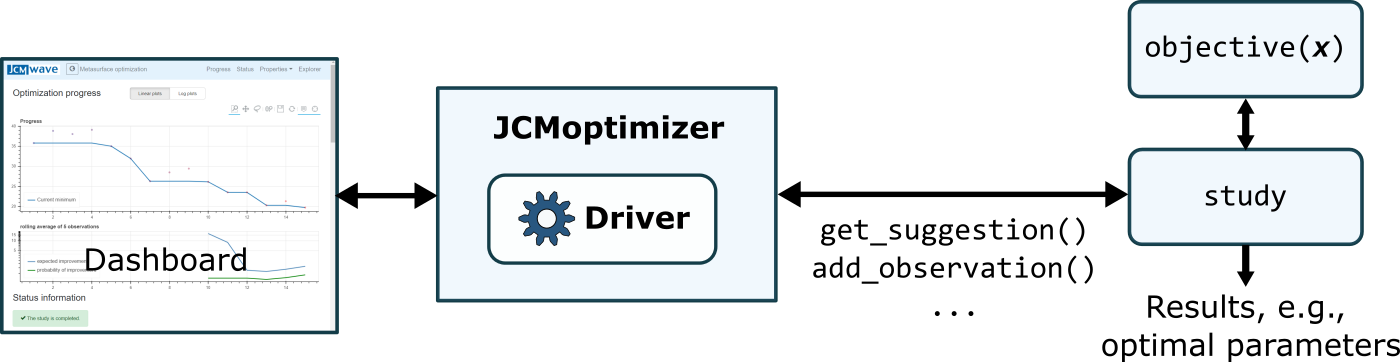# Analysis and Optimization Toolkit¶

The following section contains the documentation for using `JCMsuite`’s analysis and optimization toolkit from within Matlab.

The toolkit is provided by the server `JCMoptimizer`. All Matlab commands to interact with the `JCMoptimizer` are described in the Matlab Command Reference. The available drivers for performing various numerical studies are described in the Driver Reference. The driver reference also includes various simple Matlab code examples. A tutorial example for a parameter optimization can be found here.

The toolkit enables, for example, the efficient parameter optimization or sensitivity analysis of expensive black-box functions. Typically, the evaluation of these objective functions involves the finite-element solution of a photonic structure. However, the toolkit can be used in conjuction with any function that can be evaluated with Matlab.

A numerical study consists of retriving parameter values from the server at which the objective function is to be evaluated. The result of the evaluation is sent back to the server. After the study is finished, the results (e.g. the optimal parameter values) can be retrieved from the server. During the run of the study, the progress is vizualized on a dashboard.Schematic of the interplay between Matlab and `JCMoptimizer`.

Basic usage

Here a code with some basic principles on how to perform an optimization study:

```addpath(fullfile(getenv('JCMROOT'), 'ThirdPartySupport', 'Matlab'));

% Definition of the search domain
domain = {};
domain(1).name = 'x1';
domain(1).type = 'continuous';
domain(1).domain = [-1.5, 1.5];
domain(2).name = 'x2';
domain(2).type = 'continuous';
domain(2).domain = [-1.5, 1.5];

% Creation of the optimization study object. This will automatically
% open a browser window with a dashboard showing the optimization progress.
study = jcmwave_optimizer_create_study('domain', domain, 'name', 'basic example');
study.set_parameters('max_iter', 80);

% Definition of the objective function
objective_basic = @(x1,x2) 10*2 + (x1^2-10*cos(2*pi*x1)) + (x2^2-10*cos(2*pi*x2));

% Run the minimization
while(not(study.is_done))
sug = study.get_suggestion();
val = objective_basic(sug.sample.x1, sug.sample.x2);
obs = study.new_observation();
end
```

Extended functionality

This is an example demonstrating some extended functionality:

```addpath(fullfile(getenv('JCMROOT'), 'ThirdPartySupport', 'Matlab'));

% Definition of the search domain with continuous, discrete and fixed parameters
domain = {};
domain(1).name = 'x1';
domain(1).type = 'continuous';
domain(1).domain = [-1.5, 1.5];
domain(2).name = 'x2';
domain(2).type = 'continuous';
domain(2).domain = [-1.5, 1.5];
domain(3).name = 'x3';
domain(3).type = 'discrete';
domain(3).domain = [-1,0,1];
domain(4).type = 'fixed';
domain(4).domain = 2;

% Definition of a constraint on the search domain
constraints = {};
constraints(1).name = 'circle';
constraints(1).constraint = 'sqrt(x1^2 + x2^2) - radius';

% Creation of the optimization study with a predefined study_id and
% path save_dir where the information of optimization runs are stored
% under the name [study_id '.jcmo'].
save_dir = tempdir;
study = jcmwave_optimizer_create_study('domain',domain, 'constraints',constraints, ...
'name','Extended example', 'study_id', ...
'extended_example', 'save_dir',save_dir);

% The objective function is defined in an external file objective.m
% Sometimes some objective function values are known, e.g. from
% previous calculations. These can be added manually to speed up
% the optimization.
sample = struct('x1',0.7,'x2',0.6,'x3',0.0);
obs = objective(sample);

% Run 2 objective function evaluations in parallel
% Do the first hyperparameter optimization only after 30 observations
num_parallel = 2;
study.set_parameters('num_parallel', num_parallel, ...
'min_PoI', 1e-5, 'optimization_step_min', 30);

% Sometimes the evaluation of the objective function fails. Since the
% corresponding observation cannot be added, the suggestion has to be removed
% from the study
suggestion = study.get_suggestion();
study.clear_suggestion(suggestion.id, 'Evaluation failed');

% The objective evaluations are performed in parallel.
% If an evaluation fails, the corresponding suggestion is cleared.
while(not(study.is_done))
parfor ii = 1:num_parallel
sug = study.get_suggestion();
obs = objective(sug.sample);
end
end

% Now the found minimum is converged via gradient descendent (L-BFGS-B)
info = study.info();
sample = info.min_params;
study2 = jcmwave_optimizer_create_study('study_id', study.id, 'save_dir',save_dir,...
'name','Extended example - phase 2', 'driver','L_BFGS_B_Optimization');

% The single minimizer (num_initial=1) stops if the stepsize (eps) is below 1e-9
% By setting max_num_minimizers to 1, one prevents that the minimizer restarts at a
% different position after convergence
study2.set_parameters('num_initial',1,'eps',1e-9,'max_num_minimizers',1,...
'jac',true,'initial_samples',{sample});

while(not(study2.is_done))
sug = study2.get_suggestion();
obs = objective(sug.sample);
end

%plot the optimization progress
data = study2.get_data_table();
info = study2.info();
semilogy(data.iteration,data.cummin);
title(sprintf('Minimum found at (%0.3e, %0.3e, %d)',info.min_params.x1, info.min_params.x2, ...
info.min_params.x3))
xlabel('iterations')
ylabel('minimal objective value')
grid on

%delete results file
delete(fullfile(save_dir,'extended_example.jcmo'));
```